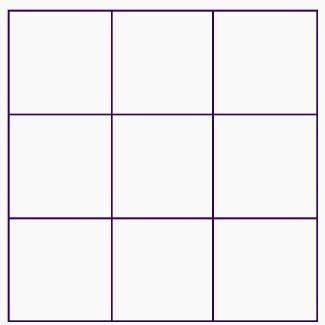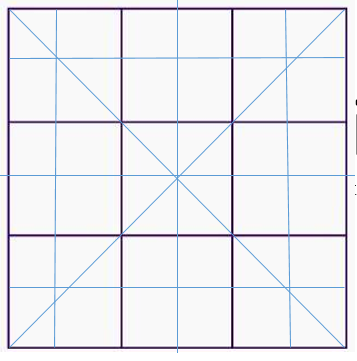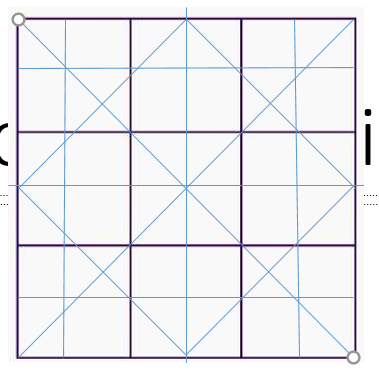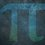# Magic Square Connections Study

Now this is for $$n \geq 2$$ as $$n = 1$$ yields no connections.

Study:

Imagine a $3 \times 3$ magic square without numbers:Now, in a magic square, you need to make sure all the rows, columns and (sometimes) diagonals add up to one number.

So:Most people would see $8$ connections in this picture. But I see differently. I see $12$ connections:Why $12$? Why not see as everybody else?

Well, from what normal people see, the amount of connections in a magic square forms this arithmetic sequence:

$0, 6, 8, 10, 12, 14, ...$

There's no $n$th term.

But, from my perspective:

$0, 6, 12, 18, 24, 30$

There's a $n$th term for this sequence: $6n - 6$.

And there is no additional connections.

Also, when $n \geq 10$, it gets harder to draw a magic square with all the connections, so we need an algebraic approach.

What people normally see is $2n + 4$ (excluding the $0$).

However, the ratio $\frac{6n - 6}{2n + 4}$ shows, the amount of magic square connections missing gets larger with each increase of $1$ to $n$.

Also, the amount of triangles increases at a geometric rate:

In my square above, you should see (forgive me for the messy lines - it was done on PowerPoint) $16$ triangles. (If you can't see $16$ triangles, don't worry - I first did this study on paper, so I know exactly how many are there.)

Continuing the sequence in both directions, we obtain:

$0, 4, 16, 36, 64, 100, ...$

The $n$th term is: (oddly, $2n^2$ or $(2n)^2$ don't match the exact sequence.) $(n - 1(4(n - 1))$.

Note that this $n$th term is from my perspective.

Now, imagine functions $M_S(A)$ and $M_S(P)$ (where$M_S$ denotes the magic square, $(A)$ denotes area and $(P)$ denotes perimeter):

$M_S(A) = n^2$

$M_S(P) = 4n$

The ratios are:

$P : A =$ $\frac{n}{4}$

$A : P =$ $\frac{4}{n}$

Based on these facts:

$P : A = (A : P)^{-1}$

$A : P = (P : A)^{-1}$

Now, if we divide the amount of triangles by the amount of connections ($C : N_T$ for short), we get: $\frac{4(n - 1)}{6}$

Inverting it gives us the amount of connections divided by the amount of triangles: $\frac{6}{4(n - 1)}$

So:

$N_T : C = (C : N_T)^{-1}$

$C : N_T = (N_T : C)^{-1}$

Now, showing a $1 \times 1$ square and increasing $n$ by $1$ gives us this:

$1, 3, 5, 7, 9, ...$

The $n$th term for this sequence is $2n - 1$ - this $n$th term denotes the function $A_D(x)$ (where $A_D$ is the amount of dots.)

Also, since a square's rotational symmetry is $4$, a magic square with all the connections will also have a rotational symmetry of $4$.

Now all of this information is useful for solving questions like this:

Example $1$:

You have a $n \times n$ square.

You have one clue:

$n - 8 =$$\frac{n}{9}$

Find the true amount of connections, the amount of dots, the amount of triangles and add them together.

Solution:

$n - 8 =$$\frac{n}{9}$

$9(n - 8) = n$

$9n - 72 = n$

$8n - 72 = 0$

$8n = 72$

$n = 9$

Substitute $n = 9$ into:

$(n - 1(4(n - 1)))$ - $256$

$6n - 6$ - $48$

$2n - 1$ - $17$

$256 + 48 + 17 = \fbox{321}$

Now, to the last BRILLIAthon problem:

How many connections are there in a $20 \times 20$ square:

Solution:

$(6 \times 20) - 6 = 6 \times 19 = \fbox{114}$

I hope you enjoyed this study and learnt something from this!Note by Yajat Shamji
1 year ago

This discussion board is a place to discuss our Daily Challenges and the math and science related to those challenges. Explanations are more than just a solution — they should explain the steps and thinking strategies that you used to obtain the solution. Comments should further the discussion of math and science.

When posting on Brilliant:

• Use the emojis to react to an explanation, whether you're congratulating a job well done , or just really confused .
• Ask specific questions about the challenge or the steps in somebody's explanation. Well-posed questions can add a lot to the discussion, but posting "I don't understand!" doesn't help anyone.
• Try to contribute something new to the discussion, whether it is an extension, generalization or other idea related to the challenge.

MarkdownAppears as
*italics* or _italics_ italics
**bold** or __bold__ bold
- bulleted- list
• bulleted
• list
1. numbered2. list
1. numbered
2. list
Note: you must add a full line of space before and after lists for them to show up correctly
paragraph 1paragraph 2

paragraph 1

paragraph 2

[example link](https://brilliant.org)example link
> This is a quote
This is a quote
    # I indented these lines
# 4 spaces, and now they show
# up as a code block.

print "hello world"
# I indented these lines
# 4 spaces, and now they show
# up as a code block.

print "hello world"
MathAppears as
Remember to wrap math in $$ ... $$ or $ ... $ to ensure proper formatting.
2 \times 3 $2 \times 3$
2^{34} $2^{34}$
a_{i-1} $a_{i-1}$
\frac{2}{3} $\frac{2}{3}$
\sqrt{2} $\sqrt{2}$
\sum_{i=1}^3 $\sum_{i=1}^3$
\sin \theta $\sin \theta$
\boxed{123} $\boxed{123}$

Sort by:

@Levi Walker, check out my study!

First ever in my profile!

- 1 year ago

Really nice! It seems that with more connections they would also lose their "magic" property where summing along a connection will lead to the same constant.

- 1 year ago

I know. There is only one way that the ''magic'' property would stay (investigated this as well):

Putting $0$ in every square!

- 1 year ago

I think you should be more clear on what "connections" means. I did not know what you meant in the problem, and I had to not do your problem because of that.

- 1 year ago

Well, I think you understand now, right?

- 1 year ago

No, not really.

- 1 year ago